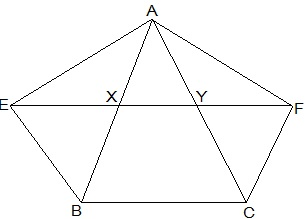Guru

# XY is a line parallel to side BC of a triangle ABC. If BE || AC and CF || AB meet XY at E and F respectively, show that ar(ΔABE) = ar(ΔACF) Q.8

• 0

Yesterday i was doing the question from class 9th ncert book of math of Areas of Parallelograms and Triangles chapter of exercise 9.3  What is the easiest way for solving it because i was not able to do this question please help me for solving this question XY is a line parallel to side BC of a triangle ABC. If BE || AC and CF || AB meet XY at E and F respectively, show that ar(ΔABE) = ar(ΔACF)

Share

1.Given,

XY || BC, BE || AC and CF || AB

To show,

ar(ΔABE) = ar(ΔAC)

Proof:

BCYE is a || gm as ΔABE and ||gm BCYE are on the same base BE and between the same parallel lines BE and AC.

∴,ar(ABE) =  ½ ar(BCYE) … (1)

Now,

CF || AB and XY || BC

⇒ CF || AB and XF || BC

⇒ BCFX is a || gm

As ΔACF and || gm BCFX are on the same base CF and in-between the same parallel AB and FC .

∴,ar (ΔACF)= ½  ar (BCFX) … (2)

But,

||gm BCFX and || gm BCYE are on the same base BC and between the same parallels BC and EF.

∴,ar (BCFX) = ar(BCYE) … (3)

From (1) , (2) and (3) , we get

ar (ΔABE) = ar(ΔACF)

⇒ ar(BEYC) = ar(BXFC)

As the parallelograms are on the same base BC and in-between the same parallels EF and BC–(iii)

Also,

△AEB and ||gm BEYC are on the same base BE and in-between the same parallels BE and AC.

⇒ ar(△AEB) = ½ ar(BEYC) — (iv)

Similarly,

△ACF and || gm BXFC on the same base CF and between the same parallels CF and AB.

⇒ ar(△ ACF) = ½ ar(BXFC) — (v)

From (iii), (iv) and (v),

ar(△ABE) = ar(△ACF)

• 0
• Share